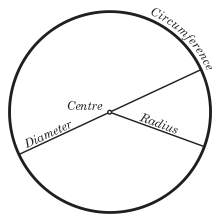# Disk (mathematics)

﻿
Disk (mathematics)A disk is the region bounded by a circle. An open disk is the interior of the disk excluding the bounding circle, while a closed disk (see closed set) is the open disk together with the bounding circle.

In geometry, a disk (also spelled disc) is the region in a plane bounded by a circle.

A disk is said to be closed or open according to whether or not it contains the circle that constitutes its boundary. In Cartesian coordinates, the open disk of center (a,b) and radius R is given by the formula$D=\{(x, y)\in {\mathbb R^2}: (x-a)^2+(y-b)^2 < R^2\}$

while the closed disk of the same center and radius is given by$\overline{ D }=\{(x, y)\in {\mathbb R^2}: (x-a)^2+(y-b)^2 \le R^2\}.$

The area of a closed or open disk of radius R is πR2 (see π).

The ball is the disk generalised to metric spaces. In context, the term ball may be used instead of disk.

In theoretical physics a disk is a rigid body which is capable of participating in collisions in a two-dimensional gas. Usually the disk is considered rigid so that collisions are deemed elastic.

## Geometry

The Euclidean disk is circular symmetrical.

## Topological notions

The open disk and the closed disk are not homeomorphic, since the latter is compact and the former is not. However from the viewpoint of algebraic topology they share many properties: both of them are contractible and so are homotopy equivalent to a single point. This implies that their fundamental groups are trivial, and all homology groups are trivial except the 0th one, which is isomorphic to Z. The Euler characteristic of a point (and therefore also that of a closed or open disk) is 1.

Every continuous map from the closed disk to itself has at least one fixed point (we don't require the map to be bijective or even surjective); this is the case n=2 of the Brouwer fixed point theorem. The statement is false for the open disk: consider for example$f(x,y)=\left(\frac{x+\sqrt{1-y^2}}{2},y\right)$

which maps every point of the open unit disk to another point of the open unit disk slightly to the right of the given one.

Wikimedia Foundation. 2010.

### Look at other dictionaries:

• Mathematics and Physical Sciences — ▪ 2003 Introduction Mathematics       Mathematics in 2002 was marked by two discoveries in number theory. The first may have practical implications; the second satisfied a 150 year old curiosity.       Computer scientist Manindra Agrawal of the… …   Universalium

• mathematics — /math euh mat iks/, n. 1. (used with a sing. v.) the systematic treatment of magnitude, relationships between figures and forms, and relations between quantities expressed symbolically. 2. (used with a sing. or pl. v.) mathematical procedures,… …   Universalium

• Mathematics of radio engineering — A complex valued function. The mathematics of radio engineering is a pleasant and very useful subject. This article is an attempt to provide a reasonably comprehensive summary of this almost limitless topic. While the ideas have historically… …   Wikipedia

• List of mathematics articles (D) — NOTOC D D distribution D module D D Agostino s K squared test D Alembert Euler condition D Alembert operator D Alembert s formula D Alembert s paradox D Alembert s principle Dagger category Dagger compact category Dagger symmetric monoidal… …   Wikipedia

• Ball (mathematics) — N ball redirects here. For the video game, see N ball (game). A ball is the inside of a sphere In mathematics, a ball is the space inside a sphere. It may be a closed ball (including the boundary points) or an open ball (excluding them). These… …   Wikipedia

• Area of a disk — Part of a series of articles on the mathematical constant π …   Wikipedia

• List of mathematics articles (U) — NOTOC U U duality U quadratic distribution U statistic UCT Mathematics Competition Ugly duckling theorem Ulam numbers Ulam spiral Ultraconnected space Ultrafilter Ultrafinitism Ultrahyperbolic wave equation Ultralimit Ultrametric space… …   Wikipedia

• Unit disk — For other uses, see Disc (disambiguation). An open Euclidean unit disk In mathematics, the open unit disk (or disc) around P (where P is a given point in the plane), is the set of points whose distance from P is less than 1 …   Wikipedia

• Unit disk graph — In geometric graph theory, a unit disk graph is the intersection graph of a family of unit circles in the Euclidean plane. That is, we form a vertex for each circle, and connect two vertices by an edge whenever the corresponding circles cross… …   Wikipedia

• List of mathematics articles (P) — NOTOC P P = NP problem P adic analysis P adic number P adic order P compact group P group P² irreducible P Laplacian P matrix P rep P value P vector P y method Pacific Journal of Mathematics Package merge algorithm Packed storage matrix Packing… …   Wikipedia